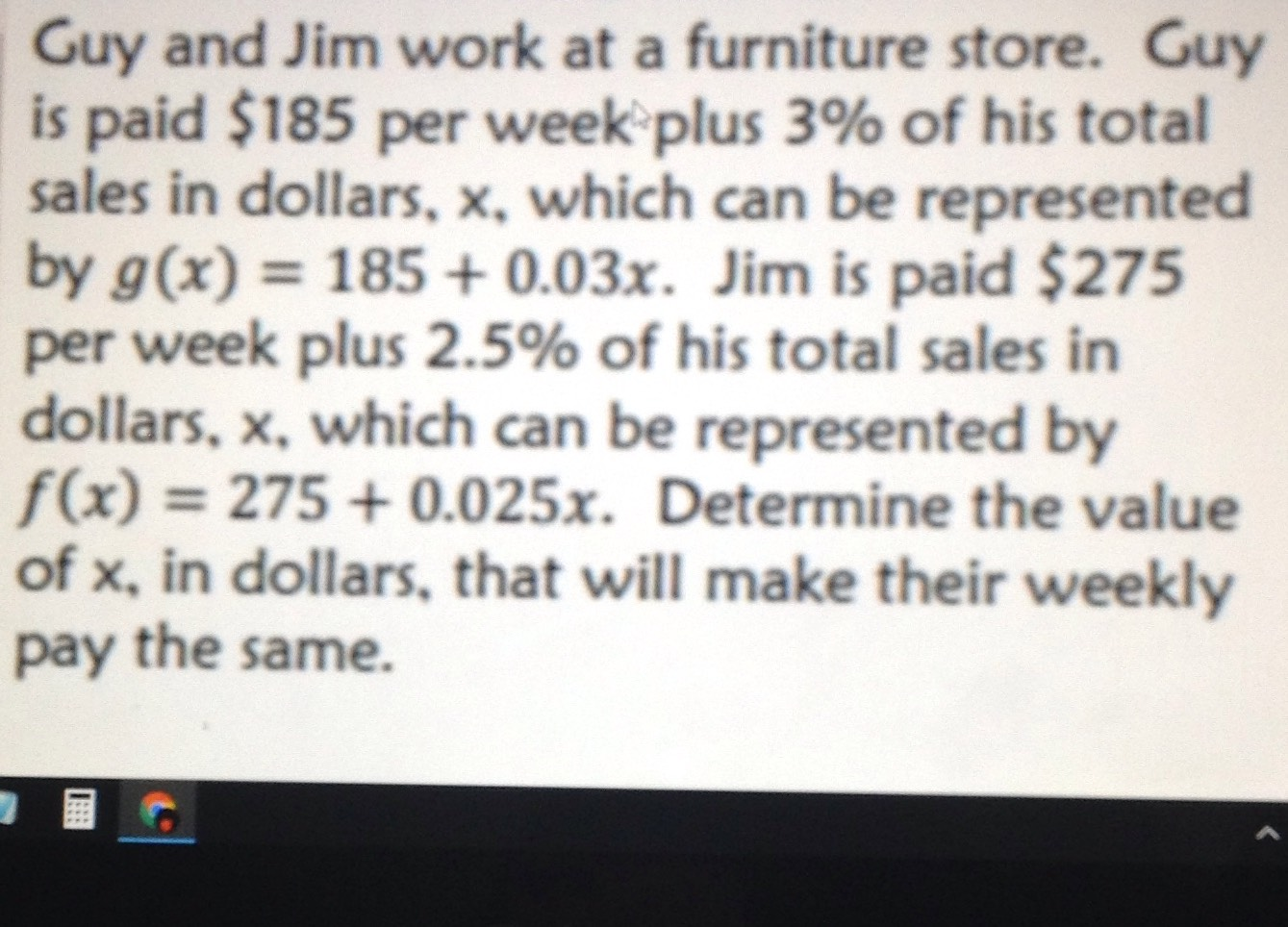### Still have math questions?

Algebra
QuestionGuy and Jim work at a furniture store. Guy is paid $$\ 185$$ per week plus $$3 \%$$ of his total sales in dollars, $$x$$ , which can be represented by $$g ( x ) = 185 + 0.03 x$$ . Jim is paid $$\ 275$$

per week plus $$2.5 \%$$ of his total sales in dollars, $$x$$ , which can be represented by

$$f ( x ) = 275 + 0.025 x$$ . Determine the value of $$x$$ , in dollars, that will make their weekly pay the same.